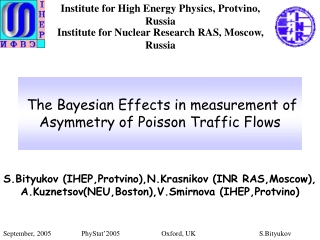DownloadDownload PresentationThe Bayesian Effects in measurement of Asymmetry of Poisson Traffic Flows

# The Bayesian Effects in measurement of Asymmetry of Poisson Traffic Flows

Download Presentation## The Bayesian Effects in measurement of Asymmetry of Poisson Traffic Flows

- - - - - - - - - - - - - - - - - - - - - - - - - - - E N D - - - - - - - - - - - - - - - - - - - - - - - - - - -
##### Presentation Transcript

1. Institute for High Energy Physics, Protvino, Russia The Bayesian Effects in measurement of Asymmetry of Poisson Traffic Flows Institute for Nuclear Research RAS, Moscow, Russia S.Bityukov (IHEP,Protvino),N.Krasnikov (INR RAS,Moscow), A.Kuznetsov(NEU,Boston),V.Smirnova (IHEP,Protvino) September, 2005 PhyStat’2005 Oxford, UK S.Bityukov

2. Introduction Different spin effects remain one of the most mysterious phenomena in particle physics today, in particular, one-spin asymmetry. During the last 20 years these effects are studied in experiments at CERN, SLAC and Protvino and asymmetry of meson formations (reaching 30 %) was observed. We want to call attention to some systematic bias in measurements of the asymmetry and in estimations of the asymmetry error. The measurement and account for difference between real asymmetry and observed asymmetry are important in the planning of spin experiments and interpretation of the experimental results. In the report the using of properties statistically dual distributions (Poisson and Gamma) and of concept “confidence density of parameter” allows to show the presence of bias in reconstruction of the initial asymmetry, which produce observed asymmetry. September, 2005 PhyStat’2005 Oxford, UK S.Bityukov

3. What we keep in mind under asymmetry 1) Asymmetry(initial)- the difference between the expected number of events (m1, m2) of two different Poisson streams of events 2) Observed asymmetry - the difference between theobserved number of events (n1, n2) in Poisson streams of events September, 2005 PhyStat’2005 Oxford, UK S.Bityukov

4. The interrelation between Gamma and Poisson distributions Iff(n;m)describes the Poisson distribution of probabilities and g(m;n)is the density of Gamma-distribution G1,1+nthen (Eq.1) where and n is the observed number of casual events appearing in Poisson flow for certain period of time. This identity shows that in our case the distribution of the probability of a true value of Poisson distribution parameter (the confidence density)for observed value nis the Gamma-distribution with mode nand mean value n+1,i.e. observed value n corresponds to the most probable value of parameter. Note, that the mean value of the reconstructed confidence density of parameter is n+1 September, 2005 PhyStat’2005 Oxford, UK S.Bityukov

5. Expected bias in the measurement of asymmetry Usually, we try to estimate the mean value of parameter. This shift in determination of the mean value of Poisson distribution parameter indicates that observed asymmetry will have bias too, i.e.here is possible the approximate correction It follows from the given identity (Eq.1) we can mix Bayesian (g(m;n)) and frequentist (f(n;m)) probabilities. Also, in the case of single observation the identity (Eq.1) does not leave a place for any prior except uniform. [NIM, 502(2003)795; JHEP 09(2002)060; physics/0403069] We can estimate the scale of the possible bias in the measurement of asymmetry by Monte Carlo experiment. September, 2005 PhyStat’2005 Oxford, UK S.Bityukov

6. The arrangement of measurements We reconstruct the confidence density of parameter “initial asymmetry” by using Monte Carlo calculations. We carried out the uniform scanning of the value of asymmetry A with step 0.01 from -1 up to 1, playing with the two Poisson distributions (with parameters m1 and m2) 30000 trials for each value A with the using of function RNPSSN. m1 and m2obey the condition m1+m2= const. After performing of this experiment we have the distribution of conditional probability of true value of asymmetry (the confidence density of asymmetryA) under observed value September, 2005 PhyStat’2005 Oxford, UK S.Bityukov

7. The arrangement of measurements In Fig.1 and Fig.2 are shown the observed values of asymmetry for two values of initial asymmetry A=0 and A=0.5 . Fig.1Fig.2 September, 2005 PhyStat’2005 Oxford, UK S.Bityukov

8. The arrangement of measurements Fig.3 Observed asymmetry versusinitial asymmetry September, 2005 PhyStat’2005 Oxford, UK S.Bityukov

9. Let us fix the observed value of asymmetry and consider how is distributed the contribution of different values of A.It is shown in Fig.4 and Fig.5 for m1 +m2=100 and in Fig.6 for the case m1 +m2=10. As seen the mean value of parameter Awhich can produce the observed value of asymmetry has the bias, i.e. <A>=0.9796 (Fig.5) and <A>=0.8004 (Fig.6). The analysis of results (I) Fig.4 Fig.5 Fig.6 September, 2005 PhyStat’2005 Oxford, UK S.Bityukov

10. In Fig.7 one can see the behavior of this bias on value of observed asymmetry. As it shown in Fig.8 the resolution of the determination of the A has dependence onthe observed value of asymmetry. Fig.7 Fig.8 The analysis of results (II) September, 2005 PhyStat’2005 Oxford, UK S.Bityukov

11. Conclusion 1) The Monte Carlo experiment confirms the presence of the bias in the determination of expected asymmetry by the using of the observed asymmetry. 2) The resolution in the determination of the expected asymmetry has dependence on the value of the observed asymmetry It is conclusion that the using of concept “confidence density of parameter” gives a convenient tool for statistical analysis. The given approach allows to consider conditional distribution of the parameter for statistical inferences in Bayesian sense. September, 2005 PhyStat’2005 Oxford, UK S.Bityukov